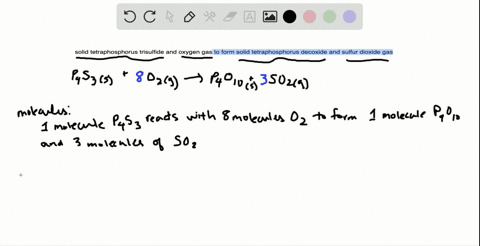Black Friday is Here! Start Your Numerade Subscription for 50% Off!Join Today### 3.116 For the reaction between solid tetraphospho…

09:32University of Maine
Problem 115

# In each pair, choose the larger of the indicated quantities or state that the samples are equal:(a) Entities: 0.4 mol of $\mathrm{O}_{3}$ molecules or 0.4 $\mathrm{mol}$ of $\mathrm{O}$ atoms(b) Grams: 0.4 $\mathrm{mol}$ of $\mathrm{O}_{3}$ molecules or 0.4 $\mathrm{mol}$ of $\mathrm{O}$ atoms(c) Moles: 4.0 $\mathrm{g}$ of $\mathrm{N}_{2} \mathrm{O}_{4}$ or 3.3 $\mathrm{g}$ of $\mathrm{SO}_{2}$(d) Grams: 0.6 $\mathrm{mol}$ of $\mathrm{C}_{2} \mathrm{H}_{4}$ or 0.6 $\mathrm{mol}$ of $\mathrm{F}_{2}$(e) Total ions: 2.3 $\mathrm{mol}$ of sodium chlorate or 2.2 $\mathrm{mol}$ of magnesiumchloride(f) Molecules: 1.0 $\mathrm{g}$ of $\mathrm{H}_{2} \mathrm{O}$ or 1.0 $\mathrm{g}$ of $\mathrm{H}_{2} \mathrm{O}_{2}$(g) Grams: $6.02 \times 10^{23}$ atoms of $^{235} \mathrm{U}$ or $6.02 \times 10^{23}$ atoms of $^{238} \mathrm{U}$

## Discussion

You must be signed in to discuss.

## Video Transcript

to compare quantities, we need to know several different relationships between moles, molecules, atoms and ions. So if you are given a certain quantity of moles, we know that every one mole of a substance contained 6.2 times 10 to the 23rd things, which is sometimes called an entity. So in this case, if we have 0.4 moles of 03 4.4 moles of 02 they both have the same number of particles, so these quantities are equal. If instead of asking about number of particles or entities, we asked about Gramm's. We need to use the molar Mass to compare. So if I have 0.4 moles of 03 to change that two grams, I use the molar Mass or looking on the periodic table, I add up. We're multiplying this case three times the molar mass of oxygen, so 0.4, divided by three times 15.99999 equals 19.2 grams. Similarly, if we look at our mules of oxygen Adams one mole has a mass of 15.999 grams, 4.4 divided by 15.999 is 6.4 grabs. So using the molar mass, we can see that 0.4 moles 03 has more grams in 0.4 moles of Oh, you can see a similar thing with different compounds four grams of end to 04 changing two moles again, I use the molar mass, which is two times the molar mass of nitrogen, plus four times the molar mass of oxygen or four. Divided by this number is 0.0 for three Knowles. 3.3 grams of eso to divided by its smaller mass, which is the molar mass sulfur plus two times the molar mass oxygen equals 0.52 moles. So we see that 3.3 grams eso to has more moles than four grams of end to four given mole to convert to grams a similar process using molar Mass. Except in this case, we multiply by the molar Mass. So 0.6 malls of C two H four times the molar mass, which is two times the molar mass of kurban, plus four times the molar mass of hydrogen tells us that this is equivalent 16.8 grams 0.6 moles of F two multiplied by its smaller mass just to times 18 0.99 eight is equivalent to 22.8 grams. So 0.6 moles of F two is more grams than 0.6 moles of C two h four. If you're given an Ionic compound and asked to look at number of ions first, we have to figure out which ions make these compounds. It's a sodium chlorate is made up of this sodium ion, an A plus in the chlorate and I in Cielo three minus. So there's one eye on a sodium in one eye on a quarry quarry to make so didn't glory. So if I have to 0.3 moles of N a. C l 03 this is equivalent to multiplying it by 6.2 times 10 to the 23rd Formula. Unit Formula Unit is just one ionic compound unit. Her mole and I know that there are two ions for every formula unit, so multiplying 2.3 times 6.2 times 10 to the 23rd times two tells us there 2.77 times 10 to the 24th ions. Similarly, with magnesium chloride magnesium is made up of M G two plus and C L minus, So the formula is mg seal, too. There are three ions, one magnesium and two Korans. So if I have 2.2 moles of magnesium chloride, changing that to formula units and then multiplying by three ions for every formula unit, this equals 3.97 times 10 to the 24 I on. So there are more total ions and 2.2 moles magnesium chloride, every look at number of molecules. In a certain quantity, you have to change from grams to moles to molecules, So one gram of H 20 It's changed two moles by dividing by the molar mass, which is two times molar mass of hydrogen, plus the molar mass of oxygen, then changing two molecules by multiplying by, Ah, for God, rose number. Similarly, the same steps for H 202 or hydrogen peroxide. The only difference is the molar mass. One more is equal to two times the molar mass of hydrogen, plus two times the molar mass of oxygen and then multiplying again by Abu God. Rose number or 1.77 times 10 to the 22nd molecules, so there are more molecules in one gram of water. Then there are in one gram of hydrogen peroxide. And finally, if we're given an atomic symbol, you to 35. This means that the atomic weight is 235 atomic mass units, as opposed to a mole of uranium to 38. And so this has a slightly larger mass than this. So subsequently you to 38 we'll have more mass than you 2 35